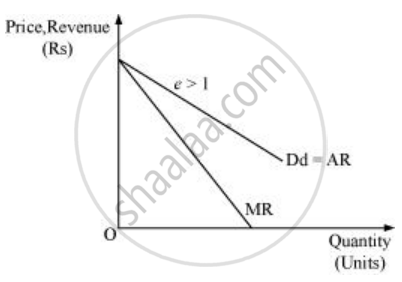Share

# What is the Value of the Mr When the Demand Curve is Elastic? - Economics

ConceptOther Non - Perfectly Competitive Markets

#### Question

What is the value of the MR when the demand curve is elastic?

#### Solution

When demand curve is elastic (ed > 1), then according to the relationship MR =P(1-1/e_d) , the fraction 1/e_d will be less than 1.

Hence, MR will be positive when P (1-1/e_d) is positive.Is there an error in this question or solution?

#### APPEARS IN

Solution What is the Value of the Mr When the Demand Curve is Elastic? Concept: Other Non - Perfectly Competitive Markets.
S